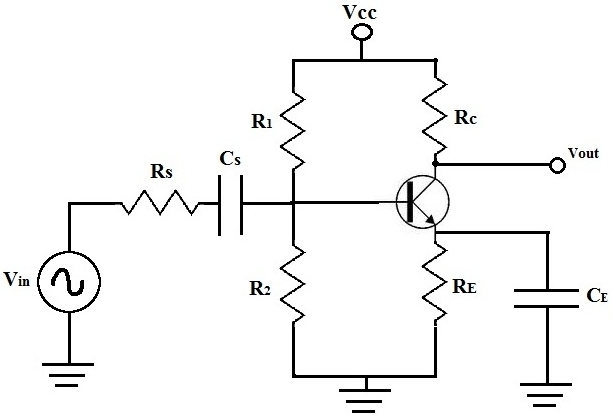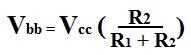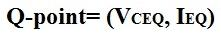﻿ DC Analysis of a Bipolar Junction Transistor Circuit

# DC Analysis of a Bipolar Junction Transistor CircuitWhat is shown above is a typical bipolar junction transistor circuit.

In this article, we're going to show how to perform DC analysis of this transistor circuit.

When doing DC analysis, all AC voltage sources are taken out of the circuit because they're AC sources. DC analysis is concerned only with DC sources. We also take out all capacitors because in DC, capacitors function as open circuits. For this reason, everything before and after capacitors are removed, which in this circuit includes resistor, Rs.

Below is the schematic of the circuit above with respective to DC analysis:Now let's do the calculations to find the Vbb, Rb, Ieq, and Vceq. From this then, we can find the quiescient or just simply Q-point of this transistor circuit.